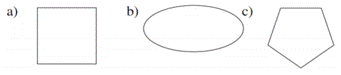Chapter 2.6, Problem 7E### Elementary Geometry for College St...

6th Edition
Daniel C. Alexander + 1 other
ISBN: 9781285195698

#### Solutions

Chapter
Section### Elementary Geometry for College St...

6th Edition
Daniel C. Alexander + 1 other
ISBN: 9781285195698
Textbook Problem
1 views

# Which geometric figures have symmetry with respect to a point?To determine

To choose:

The geometric figures having symmetry with respect to a point.

Explanation

Consider the geometric figure (a) and consider point P at the center of the geometric figure.

Figure (1)

Consider arbitrary points A, B, and C on the polygon.

Figure (2)

For each arbitrary point on the polygons, there is second point corresponding to it such that point P is the midpoint of the line formed by the points.

Figure (3)

The points A, B, and C, on the polygon corresponding to points A, B, and C on the such that P is the midpoint of the line from the points.

The geometric figure (a) is symmetric about point P.

Consider the geometric figure (b) and consider point P at the center of the geometric figure.

Figure (4)

Consider arbitrary points A, B, and C on the polygon.

### Still sussing out bartleby?

Check out a sample textbook solution.

See a sample solution

#### The Solution to Your Study Problems

Bartleby provides explanations to thousands of textbook problems written by our experts, many with advanced degrees!

Get Started

#### Find more solutions based on key concepts